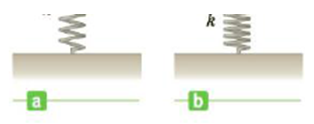Chapter 5, Problem 65AP

Chapter
Section
Textbook Problem

An archer pulls her bowstring back 0.400 m by exerting a force that increases uniformly from zero to 230 N. (a) What is the equivalent spring constant of the bow? (b) How much work does the archer do in pulling the bow?Figure P5.64

(a)

To determine
The equivalent spring constant of the bow.

Explanation

Given Info:

The length that the archer pulls back her bow-spring is 0.400m .

The force on the string is increasing uniformly from zero to 230.0N .

Formula to calculate the spring constant of the bow is,

k=Ffxf

• Ff is the final force exerted on the bow
• xf is the final length that the archer pulls back her bow-spring

Substitute 230.0N for Ff and 0

(b)

To determine
The work done by the archer did to pull the bow spring back.

Still sussing out bartleby?

Check out a sample textbook solution.

See a sample solution

The Solution to Your Study Problems

Bartleby provides explanations to thousands of textbook problems written by our experts, many with advanced degrees!

Get Started

The nervous system cannot use fat as fuel. T F

Nutrition: Concepts and Controversies - Standalone book (MindTap Course List)

What is hereditarianism, and what is the invalid assumption it makes?

Human Heredity: Principles and Issues (MindTap Course List)

Do the same types of gene control operate in bacterial cells and eukaryotic cells?

Biology: The Unity and Diversity of Life (MindTap Course List)